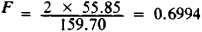# Gravimetric Analysis

Also found in: Dictionary, Thesaurus, Medical, Financial, Wikipedia.

## gravimetric analysis

[grav·ə′me·trik ə′nal·ə·səs]
(analytical chemistry)
That branch of quantitative analytical chemistry in which a desired constituent is converted, usually by precipitation or combustion, to a pure compound or element, of definite known composition, and is weighed; in a few cases a compound or element is formed which does not contain the constituent but bears a definite mathematical relationship to it.
McGraw-Hill Dictionary of Scientific & Technical Terms, 6E, Copyright © 2003 by The McGraw-Hill Companies, Inc.
The following article is from The Great Soviet Encyclopedia (1979). It might be outdated or ideologically biased.

## Gravimetric Analysis

one of the important methods of quantitative chemical analysis; based on the accurate measurement of the weight of a substance. The substance whose weight is to be determined is usually separated from the sample being analyzed in the form of a poorly soluble compound of constant, known chemical composition, since the extraction of a chemically pure element entails great difficulties and is sometimes impossible.

Gravimetric analysis begins by taking an accurately weighed amount of the sample being analyzed and dissolving it. Then, by adding the appropriate reagent, a poorly soluble precipitate of a compound is produced; it contains the element being determined. The precipitate is separated from the solution by filtration, washed, and dried or calcined to constant weight. If the weight of the sample a taken for analysis, the weight of the precipitate b, and its composition are known, the content X of the substance to be found is calculated (generally as a percentage by weight): X = a x F x 100/b, where F is a conversion factor equal to the ratio of the atomic weight of the substance to be determined (or a multiple of the latter) to the molecular weight of the compound of the precipitate. For example, when determining the amount of iron (atomic weight 55.85) separated as its oxide (molecular weight 159.70),The most important part of gravimetric analysis is the production of an easily filtrable, poorly soluble precipitate—if possible, in the form of large crystals (the loss of material caused by solubility should not exceed .1 mg)—that is free from foreign substances that are not removed by drying or calcination. Gravimetric analysis is characterized by high accuracy: experimental error does not exceed 0.1 percent, and with particularly painstaking work it may be reduced to 0.02–0.03 percent. The drawbacks of gravimetric analysis are the time required and the need to use comparatively large amounts of material for analysis (about 0.5 g). The latter disadvantage can be eliminated by using micro and ultramicro methods.

Gravimetric analysis is used to determine the chemical composition of rocks, minerals, and alloys and for quality control of raw materials and finished products in a number of branches of industry. Among the various kinds of gravimetric analysis are assay and electrochemical analysis.

### REFERENCE

Kreshkov, A. P. Osnovy analiticheskoi khimii, 3rd ed., vol. 2. Moscow. 1971.
Mentioned in ?
References in periodicals archive ?
As demonstrated in Figures 6(a) and 6(b), both CMM and Micro-CT measurement indicated higher wear compared to gravimetric analysis. With the help of web tool created by Lin and validated by Yu , the CCC, precision (Pearson correlation coefficient measuring how closely the observations deviate from the best-fit line), and accuracy (the closeness of the observations and target values in terms of both means and variances) coefficients were calculated, which are illustrated in Table 3.
Since the major effect of NaHC[O.sub.3] addition was on the total fixed solids, it could be assessed from the stoichiometric equations as a function of temperature increase during gravimetric analysis, evidenced by a satisfactory fit between the experimental and theoretical values.
Set against these problems is the fact that gravimetric analysis of the uterus sometimes also yields conflicting or weak responses, as illustrated by the results observed with the nine mouse uterotrophic assays of BPA reported by Tinwell et al.
Gravimetric analysis of the 37 millimeter (mm) diameter, 5.0 micrometer ([micro]m) pore size polyvinyl chloride (PVC) filters was performed on site.
Thermal Gravimetric Analysis describes the thermal degradation temperature of a polymer.
(4) Thermal gravimetric analysis results revealed that the sludge contained approximately 3 wt% of inorganic compound, 25 wt% of water, and 72 wt% of hydrocarbon.
Thermal gravimetric analysis was used to study the thermal stability of the polymer clay nanocomposites as shown in Fig.
In addition to the classic instruments for differential scanning calorimetry (DSC), thermal gravimetric analysis (TGA), thermo-mechanical analysis (TMA), and Dynamic Mechanical Analysis (DMA), we also offer instruments for specific applications such as melting point, dropping point, softening point, and thermal-optical analysis (TOA).
In the thermal gravimetric analysis (TGA), samples were held at 150[degrees]C for 24 hours.
The weight loss and thermal behavior of sample were also evaluated from the Thermo gravimetric analysis data by using DSC-SDT Q 600 at heating rate of 10 oC up to 1400 oC.
Figure 1 shows the thermal gravimetric analysis (TGA) of wood fiber, which is a plot of the percent weight remaining of the wood fiber sample versus temperature.
Table 1 represents the average data of gravimetric analysis of 10 stents and the Table 2 those of strut widths / width reduction of the laser cut, pickled and electropolished stent.

Site: Follow: Share:
Open / Close